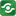@import url(http://www.google.com/cse/api/branding.css);CSGNetwork Custom SearchĀ
 CSGNetwork Snippets Calculators Top Free Apps Home Back

Right click this window and select "view source" in order to copy the source for this script.

# Line Calculators

There are two calculators here, available for lines: a distance calculator and a mid-point calculator. Learn and enjoy...

## Distance Calculator

Finds the length of a line. Just give the coordinates of the start and end points of the line.
It uses Pythagoras' formula: the square of the hypotenuse is equal to the sum of the squares on the other two sides.
D = sqr((X2-X1)2 + (Y2 - Y1)2)

( X1 , Y1 ) ( X2 , Y2 )

## Mid-Point Calculator

Consider the X values separately from the Y values. Subtract the smaller X value from the larger one to get the distance along the X axis between the two values. Now divide that by 2 to get half the distance, and add that distance back to the lower value to get the midpoint along the X axis. Now do the same for the Y values: Subtract the smaller Y value from the larger one to get the distance, get half that, and add it to the lower value.

( X1 , Y1 ) ( X2 , Y2 )